"

## Scope: Local, Global and Lexical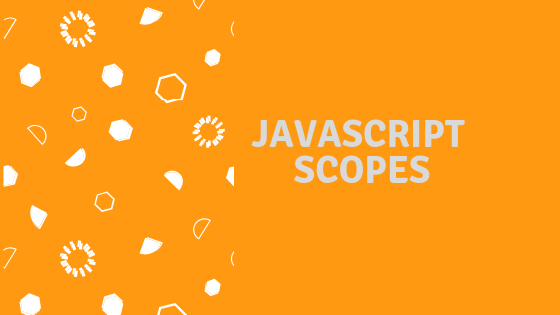A scope is like a limited area. Scopes are the essential part of every programming language. They are behaving like a limited space for a declaration i.e where every variable is declared and what part of the program that variable can be accessed.

As MDN Says — The context in which values and expressions are “visible,” or can be referenced.

There are two types of scopes:-

• Global Scope

• Local Scope But I also going to talk about Lexical Scope.

## What is Global Scope?

Global Scope is a term used when a variable is declared outside any of the functions i.e the variables should be declared globally.

``````var a = 'global';               //I am a global scope
function iAmLocal() {           //I am a global scope
console.log(a) // 'global'
}                               //I am a global scope``````

So, If you look at the above function there’s variable a is declared globally and it can be accessed inside the function. Here, iAmLocal() function created its own scope. But here’s the variable is declared in global scope. So, the point is that if we declared a variable globally it can be accessed inside a function.

Let's take another example:-

``````var a = 'global';

function iAmLocal() {
a = 'is I am local';
}

iAmLocal();

a;   //Think and let's give it a moment ??``````

So, the value of the variable should be changed or not. Let’s know it by executing it.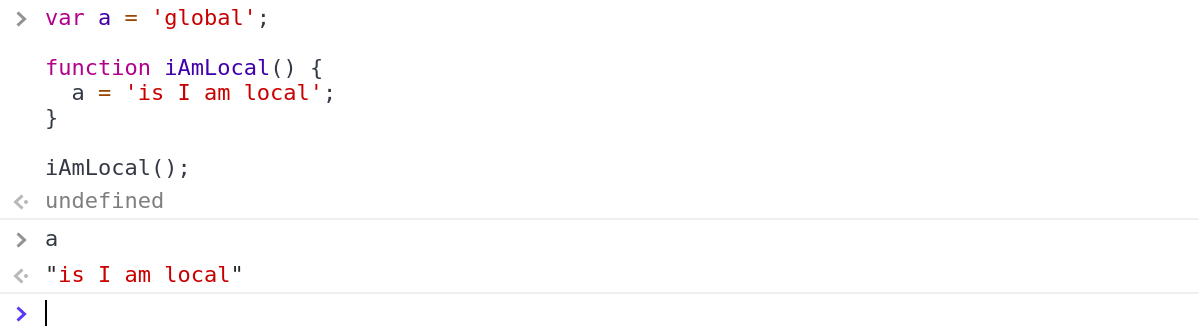It changed because the variable a is accessible inside the function because it is declared globally. So, when we call the IAmLocal() function, that reassigned the value of a again. Now, let’s go to local scope.

## What is Local Scope?

When we declare a function it creates its own scope. So, whenever a function is declared it creates its local scope which is limited to that function. So, it means that if you are declared a variable inside a function it’s limited to it. So, Let’s understand it by a code example:-

``````var a = 'global';

function iAMLocal() {
var b = 'local';
}

a; //'global'
b; //??``````

Let’s access the variable outside the function.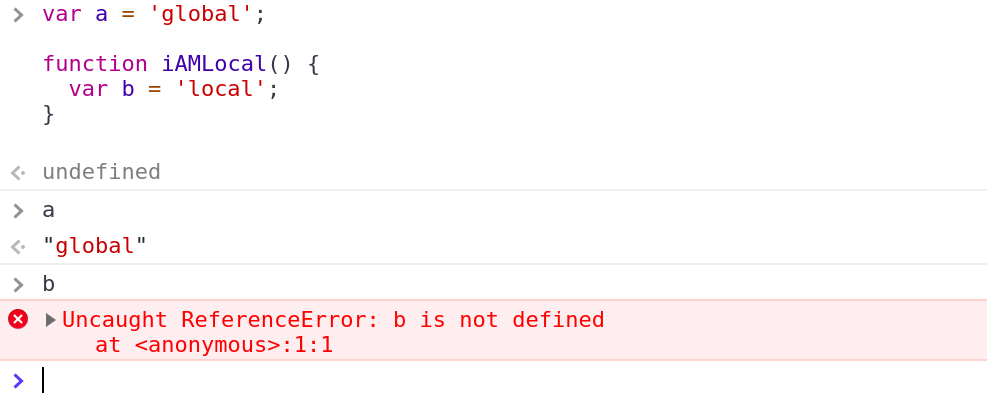OOPS!! It says an error because now the engine looks for the variable b globally but it can’t find the value because it is declared in the iAmLocal(). So, that’s why it returns an error.

Let’s take another example:-

``````function sum() {
var a = 5;
var result = 0;

// Declaring second function
function secondNumber() {
var b = 10;
return a+b;
}

//calling secondNumber() and storing it in a variable
var result = secondNumber();

return result;
}``````

So, can we access a inside the secondNumber(). Let’s try it by running it. If we get 15, that means we can.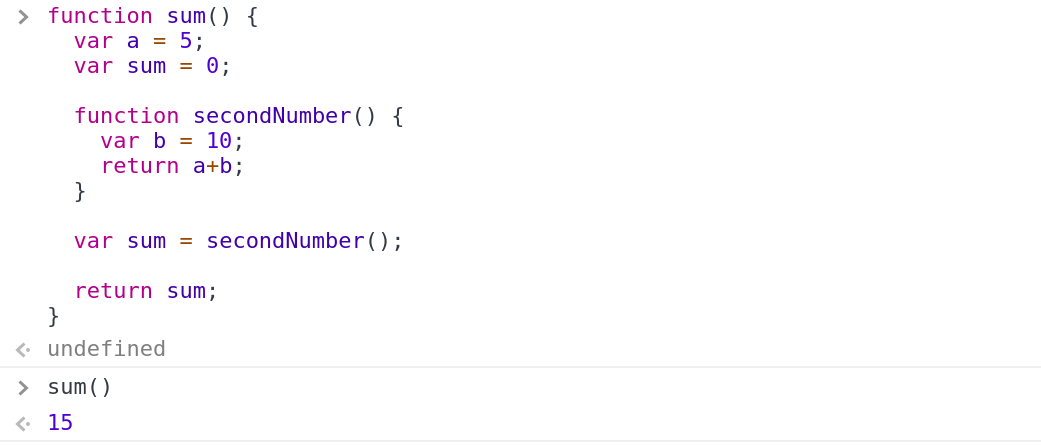Yes, It is.

## Why is that happen?

Because when sum() is declared it creates its own scope and the variables that are declared inside it can be accessible limited to the scope of the sum(). But when we declaring the secondNumber() function inside the sum() it also creates its own scope. So, when we called secondNumber() it gonna look for the value of a. It doesn’t find a it goes to it parent and checks if there’s a is not. Ya, It’s find there and execute that value successfully.

## == Lexical Scope ==

So, Lexical Scope is the same as the nested local scopes but there be little bit difference between them. In this, if the current scope doesn’t find the value then it moves outside to that scope and tries to find the value there. This process continues until it finds the value. Let’s do it by an example:-

``````var num1 = 5; //globally

var num2 = 8;  //locally

return function() {      //returning function
return num1 + num2;    //returning function
}                        //returning function
}

So, In the above code sample, I declared num1 globally because it’s outside all the loops. Now, when we declared the add() function it creates it’s own scope. That’s why num2 is declared locally so it available in the scope of the add() function. So, let’s think and look the code carefully that it gives us the 13 or not. Let’s execute it:-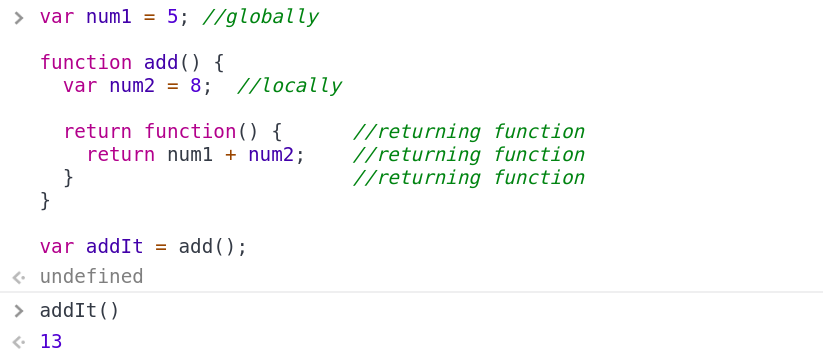So, what happened here? Let’s go on that line where we called the add() and stored this in the variable called addIt(). So, now addIt() holds the return function that is returned by the add() function and when we call the returned function we got the correct answer. So, the thing is that how can it access the value of num1. So, I write above that if the variable doesn’t find it’s value inside that function so it bubbles out i.e it goes upwards. So, now in the function that is returned by the add() function. Now, num1 finds that is my value presented in this scope if it doesn’t so it moves in the scope of the add() and checks it that my value is presented here or not. No, It goes to the global scope where it finds the value and executes it. So, the process of bubbling out and finding it’s value is called lexical scope. If it doesn’t find the value then it returns an error saying that variable is not defined.

## Let & Const -> How They Effect Scope

So, let and const are new members in the family of variables declarations in JavaScript. So, they help us to create a scope and helps the variable to not get hoisted. Let’s take an example:-

``````or(var i = 1; i <= 10; i++) {
console.log(i);
}

i;  //Can I access it out side??.``````

Let’s check out it:-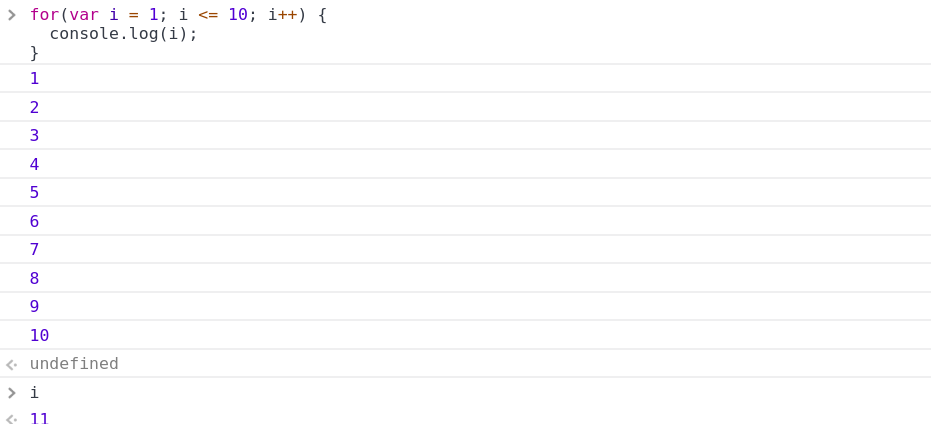So, we can access the variable i outside the loop because we know that only function can create their own scope. So, for loop declared in the global scope. That’s why in the last when the loop got executed we got the increased value of i. Let’s do it again but put let instead of var :-

``````for(let i = 1; i <= 10; i++) {
console.log(i);
}

i; //Can I access i now??``````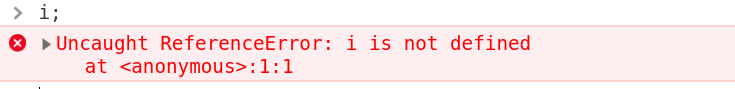Ya, it said a error saying *i is not defined* because let creates a scope to that variable. const is also same as the let but you can’t reassign a value again. So, assigning value with var, let and const depends on your need that you want to access that variable locally or want to update the variable as value reassigned to it.